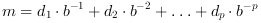Scilab Home page | Wiki | Bug tracker | Forge | Mailing list archives | ATOMS | File exchange
Change language to: Français - Português - 日本語 - Русский

See the recommended documentation of this function

Scilab Help >> Elementary Functions > Floating point > number_properties

# number_properties

determine floating-point parameters

### Calling Sequence

`pr = number_properties(prop)`

### Arguments

prop

string

pr

real or boolean scalar

### Description

This function may be used to get the characteristic numbers/properties of the floating point set denoted here by `F(b,p,emin,emax)` (usually the 64 bits float numbers set prescribe by IEEE 754). Numbers of `F` are of the form:

`sign * m * b^e`

`e` is the exponent and `m` the mantissa:the digits are in `[0, b-1]` and `e` in `[emin, emax]`, the number is said "normalized" if. The following may be gotten:

then `pr` is the radix `b` of the set `F`

prop = "digits"

then `pr` is the number of digits `p`

prop = "huge"

then `pr` is the max positive float of `F`

prop = "tiny"

then `pr` is the min positive normalized float of `F`

prop = "denorm"

then `pr` is a boolean (%t if denormalized numbers are used)

prop = "tiniest"

then if denorm = %t, `pr` is the min positive denormalized number else `pr` = tiny

prop = "eps"

then `pr` is the epsilon machine ( generally () which is the relative max error between a real `x` (such than `|x|` in `[tiny, huge]`) and `fl(x)`, its floating point approximation in `F`

prop = "minexp"

then `pr` is `emin`

prop = "maxexp"

then `pr` is `emax`

### Remarks

This function uses the lapack routine dlamch to get the machine parameters (the names (radix, digit, huge, etc...) are those recommended by the LIA 1 standard and are different from the corresponding lapack's ones).CAUTION: sometimes you can see the following definition for the epsilon machine :but in this function we use the traditional one (see prop = "eps" before) and soif normal rounding occurs andif not.

### Examples

```b = number_properties("radix")
eps = number_properties("eps")```